Filter Design using Windowing Techniques

# Filter Design using Windowing Techniques - Notes | Study Signals and Systems - Electronics and Communication Engineering (ECE)

 1 Crore+ students have signed up on EduRev. Have you?

Windowing

Disadvantage of F.S is abrupt truncation of FS expansion of the freq response. This truncation result in a poor convergence of the series.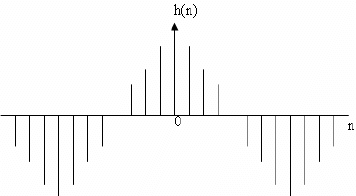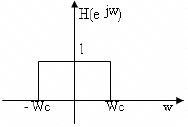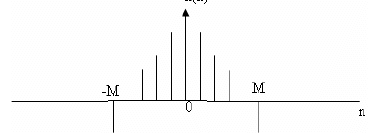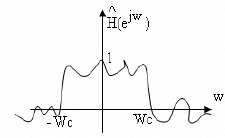The abrupt truncation of infinite series is equivalent to multiplying it with the rectangular
sequence.

WR(n) = 1        |n| ≤ M

= 0 else where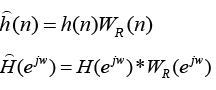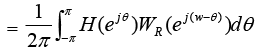WR(ejw) => FT of Rectangular Window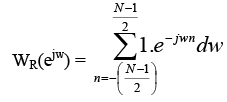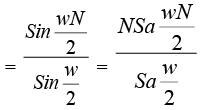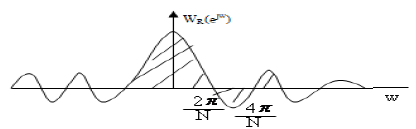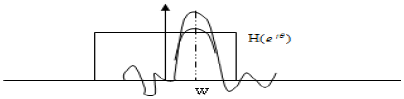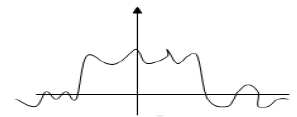•  Main lobe width =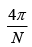& it can be reduced by increasing N, but area of side lobe will be constant.
• For larger value of N, transition region can be reduced, but we will find overshoots & undershoots on pass band and non zero response in stop band because of larger side lobes. So there overshoots and leakage will not change significantly when rectangular window is used. This result is known as Gibbs Phenomenon.

The desined window chts are

1. Small width of main lobe of the fre response of the window containing as much as of the total energy as possible.

2. Side lobes of the frequency response that decrease in energy as w tends to π

4. zero in the range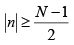Let us consider the effect of tapering the rectangular window sequence linearly from the middle to the ends.

Triangular Window: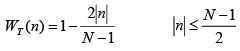= 0 else where

In this side lobe level is smaller that that of rectangular window, being reduced from -13 to -25dB to the maximum. However, the main lobe width is now 8π/N There is trade off between main lobe width and side levels.

General raised cosine window is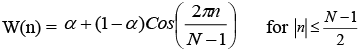= 0 else where

If α=0.5 Hanning Window

If α =0.54 Hamming Window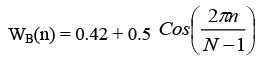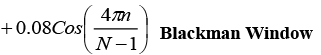Kaiser Window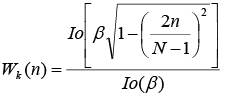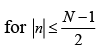= 0 else where

β is constant that specifies a freq response trade off between the peak height of the side lobe ripples and the width or energy of main lobe and Io(x) is the zeroth order modified Bessel function of the first kind. Io(x) can be computed from its power series expansion given by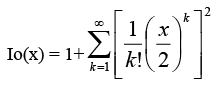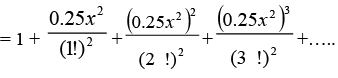Window Peak amplitude of side lobe dB Transition width of main lobe Minimum stop band deviation dB Rectangular -13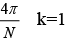-21 Triangular -25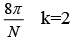-25 Hanning -31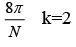-44 Hamming -41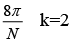-53 BlackMan -57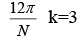-74 Kaiser variable variable -

If we let K1,W1 and K1,W1 represent cutoff (pass band) * stop band requirements for the digital filter, we can use the following steps in design procedure.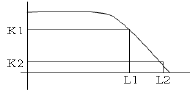1. Select the window type from table to be the one highest up one list such that the stop band gain exceeds K2.

2. Select no. of points in the windows function to satisfy the transition width for the type of window used. If Wt is the transition width, we must have Wt = W2-W1≥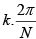where K depends on type of window used.

K=1 for rectangular , k=2 triangular…..

Therefore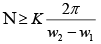If analog freq are given, it must be converted in to Digital using w= Ω T

Ex:

Apply the Hamming Window to improve the low pass filter magnitude response ontained
in ex1: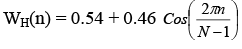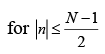= 0            else where

N = 2M+1 = 21

WH(0) = 1                 WH(6) = 0.39785

WH(1) = 0.97749       WH(7) = 0.26962

WH(2) = 0.91215       WH(8) = 0.16785

WH(3) = 0.81038       WH(9) = 0.10251

WH(4) = 0.68215      WH(10) = 0.08

WH(5) = 0.54

Next these window sequence values are multipled with coefficients h(n), obtained in ex1, to ontain modified F.S Co eff h’(n)

h’(0) =0.25

h’(1) =0.22

h’(2) =0.14517

h’(3) =0.0608

h’(4) =0

h’(5) =0.02431

h’(6) =0.02111

h’(7) =-0.0086725

h’(8) =0

h’(9) =0.00256

h’(10) =0.00255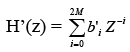bi' = h’(i-M)  0≤ i ≤ 20      h’(-n) = h’(n)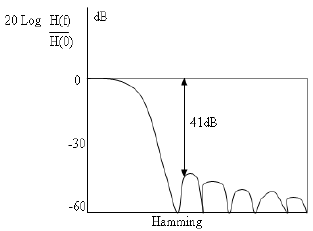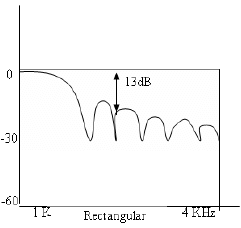Ex:

Find a suitable window and calculate the required order the filter to design a LP digital filter to be used A/D-H(Z)-D/A structure that will have a -3dB cutoff of at 30π rad/sec and an attenuation of 50dB at 45 π rad/sec. the system will use a sampling rate of 100 sample /sec

Sol:
The desired equivalent digital specifications are obtained as

Digital …..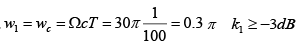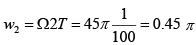k2≤ -50dB

1. to obtain a stop band attenuation of -50dB or more a Hamming window is shosen since it has the smallest transition band.

2. the approximate no. of points needed to satisfy the transition band requirement (or the order of the filter ) can be found for w1 =0.3 π rad &w2 = 0.45 π rad, using Hamming window (k=2), to be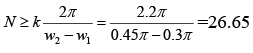N = 27 is selected

• the attractive property of the Kaiser window is that the side lobe level and main lobe width can be varied continuously by simple varying the parameter β . Also as in other window, the main lobe width can be adjusted by varying N.
• we can find out the order of Kaiser window, N and the Kaiser parameters β to design FIR filter with a pass band ripple equal to or less that Ap, a minimum stop band attenuation equal to or greater than As, and a transition width Wt, using the following steps: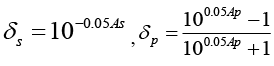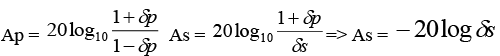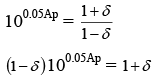Therefore: solving above eq forδ , we get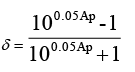Calculate As using the shosen values

Aso=-20logδ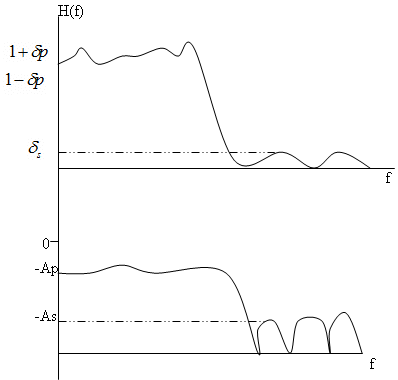Step 3:

Calculate the parameter β as follows for

β= 0            for Aso ≤ 21 dB

= 0.5842(Aso -21)0.4 + 0.07886(Aso -21)       for 21< Aso ≤ 50 dB

= 0.1102(Aso -8.7)            for Aso >50 dB

Step 4:

Calculate D as follows

D = 0.9222          for Aso≤ 21 dB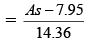for As>21 dB

Step 5:

Select the lowest odd value of N satisfying the inequality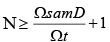Wsam : Angular Sampling frequency

Ω sam : Analog Freq

Ω t = Ω s- Ω p     for LPF

= Ω s- Ω p     for HPF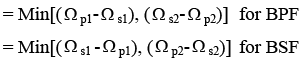-3dB cutoff freq Ω c can ve considered as follows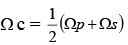for LPF & HPF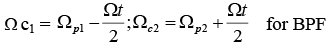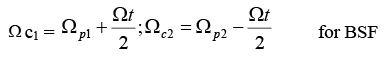Calculate the Kaiser parameter and the no. of points in Kaiser window to satisfy the following lowpass specifications.

Pass band ripple in the freq range 0 to 1.5 rad/sec ≤ 0.1 dB

Minimum stop band attenuation in 2.5 to 5.0 rad /s ≥ 40 dB

Sol:

The impulse response samples can be calculated using h(n) =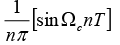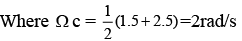And the no. of points required in this sequence can be found as follows

Step1: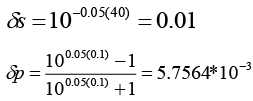Therefore we choose, δ = 5.7564*10 -3

Step 2:

Aso = -20 log( 5.7564*10-3 ) = 44.797 dB

Step 3 & 4:

β = 0.5842 ( 44.797  -21)0.4 + 0.07886 ( 44.797  -21) = 3.9524

D = 2.566

Step 5: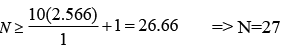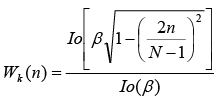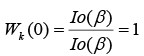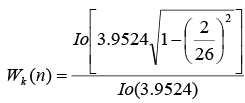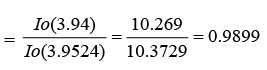The document Filter Design using Windowing Techniques - Notes | Study Signals and Systems - Electronics and Communication Engineering (ECE) is a part of the Electronics and Communication Engineering (ECE) Course Signals and Systems.
All you need of Electronics and Communication Engineering (ECE) at this link: Electronics and Communication Engineering (ECE)

## Signals and Systems

32 videos|76 docs|63 tests
 Use Code STAYHOME200 and get INR 200 additional OFF

## Signals and Systems

32 videos|76 docs|63 tests

Track your progress, build streaks, highlight & save important lessons and more!

,

,

,

,

,

,

,

,

,

,

,

,

,

,

,

,

,

,

,

,

,

;